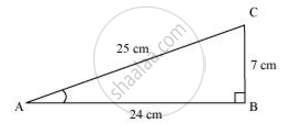# In ΔABC right angled at B, AB = 24 cm, BC = 7 m. Determine sin A, cos A - Mathematics

Sum

In ΔABC right angled at B, AB = 24 cm, BC = 7 m. Determine

sin A, cos A

#### Solution

Applying Pythagoras theorem for ΔABC, we obtain

AC2 = AB2 + BC2

= (24 cm)2 + (7 cm)2

= (576 + 49) cm2

= 625 cm2

∴ AC = sqrt(625) cm = 25 cmsin A  = ("Side opposite to" ∠A)/"Hypotenuse"="BC"/"AC"

cos A = ("Side adjacent to" ∠A)/"Hypotenuse"="AB"/"AC"=24/25

Concept: Trigonometric Ratios
Is there an error in this question or solution?
Chapter 8: Introduction to Trigonometry - Exercise 8.1 [Page 181]

#### APPEARS IN

NCERT Class 10 Maths
Chapter 8 Introduction to Trigonometry
Exercise 8.1 | Q 1.1 | Page 181
Share